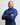# Handling Missing Data with Pandas

Academic and practice datasets tend to be in an ideal state which, is excellent for learning algorithms and quick prototyping. However, data is often incomplete or in a weird shape. Before feeding any information to machine learning algorithms, it’s a common practice and, sometimes required, to transform it into the desired state.

Usually, the first problem that we’ll have to solve during the `pre-processing` stages is the handling of missing values, in case there’s any. There are several approaches to this problem but, by far, the most common are `dropping the missing instances`, or `filling them with a computed value`.

## Dropping Entire Features(Columns)

In some occasions, our features will have so many missing values that they become unusable. In those instances, it’s safer to get rid of the entire column instead of trying to fill the gaps. When should we drop a feature? There’s no correct answer since it will depend on the specifics of the problem we aim to solve. Still, a good rule of thumb that I like to follow is throwing aways features with more than 30% of missing values. Here’s a quick way of doing just that:

``````import pandas as pd

def get_percentage_null(series):
missing = series.isnull().sum()
total = len(series)
return round(missing / total, 2)

for name, values in data_set.iteritems():
# Drops columns with over 30% missing values
percentage = get_percentage_null(data_set[name])
print("%r: %r" % (name, percentage))

if percentage >= 0.30:
print("Deleting Column %r: " % name)
data_set.drop(name, axis=1, inplace=True)``````

## Dropping Single Instances(Rows)

If we only have a handful of missing values and we can afford to lose a few entries of our data; dropping rows becomes a viable solution. Sadly, you’ll often want to hold on to as much of the data as you can, but in case you have to delete a few instances, it’s just one line of code:

``````# drops rows 5 and 10
data_set = data_set.drop([5, 10], axis=0, inplace=True)``````

In case you need help understanding the drop function, check this link.

## Filling The Gaps

A relatively common strategy is to replace missing values with statistics derived from the data. We can use the mean value of a column to fill all the empty spots or if we are concerned about outliers, the median or mode. Some domain knowledge of our data is necessary for picking a statistic that makes sense. For example, if we have a year column, it might not make sense to use the average; How do you interpret (2005 + 2006 + 2003) / 3? Things like the median and mode should be a better fit for this scenario.

Pandas has a built-in function, fillna, that does exactly what its name suggests but I prefer to wrap it around a helper function that takes care of calculation the statistics for me.

``````def fill_missing_values(data_set, column_name, strategy='custom', value=None):
# for categorical variables
if data_set[column_name].dtype == 'object':
if strategy == 'mode':
data_set[column_name].fillna(data_set[column_name].value_counts().idxmax(), inplace=True)
elif strategy == 'custom' and value is not None:
data_set[column_name].fillna(value, inplace=True)
# for numeric variables
elif data_set[column_name].dtype == 'int64' or data_set[column_name].dtype == 'float64':
if strategy == 'mode':
data_set[column_name].fillna(data_set[column_name].mode(), inplace=True)
elif strategy == 'median':
data_set[column_name].fillna(data_set[column_name].median(), inplace=True)
# custom means "whatever value you want"
elif strategy == 'custom' and value is not None:
data_set[column_name].fillna(value, inplace=True)``````

Keep in mind that this is only one of several ways of filling empty values with calculated metrics. We could also considerer a different approach like using `Linear regression` or `KNN` to compute our values. To read more click here.

## Conclusion

Most machine learning algorithms don’t work if there are missing values, thus filling or dropping them is a crucial step. However, we should be mindful of each transformation we apply to our data. Stay away from “Just do it” first ask yourself, “Does it make sense and why?” and we’ll increase our chances of using the proper values.### Randy Perez

I am a Software Developer from Santo Domingo, Dominican Republic. I have been programming for 8+ years and professionally for the last 6+ years. Currently, I work for BairesDev an international outsourcing company that lends my service to Pinterest, a social media company based in San Francisco.